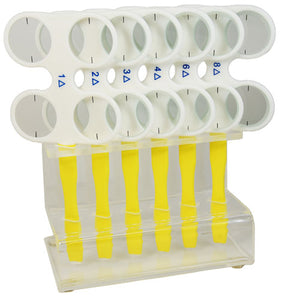# Prism Flipper Set

537900
Regular price
\$296.00
Sale price
\$296.00

## Prism Flipper Set

Prism Flipper Set with acrylic stand that contains either 5 or 6 base in and base out flippers. The 5 piece set includes one occluding flipper.

5 prism flipper set contains:

• 2 Diopter (2^+2^)= 4^ total
• 3 Diopter (3^+3^)= 6^ total
• 4 Diopter (4^+4^)= 8^ total
• 6 Diopter (6^+6^)= 12^ total
• 8 Diopter (8^+8^)= 16^ total

6 prism flipper set contains:

• 1 Diopter (1^+1^)= 2^ total
• 2 Diopter (2^+2^)= 4^ total
• 3 Diopter (3^+3^)= 6^ total
• 4 Diopter (4^+4^)= 8^ total
• 6 Diopter (6^+6^)= 12^ total
• 8 Diopter (8^+8^)= 16^ total